# Pandas Crosstab

Let’s talk about how to do some bi-variate analysis using the Crosstab function from pandas. The Crosstab function is very flexible and allows us to compare two categories.

As usual, we’ll work on this practically using a sample dataset. We’re going to analyze a BankChurn dataset to see when our customers left and the different categories for churn.

We can answer the following questions-

• What is the relationship between income and churn?
• What is the relationship between education and churn?
• What is the relationship between gender and churn?
• What income range has a higher likelihood of churn?
• Of those who churned, what is the average number of months on book by card type?

So let’s get started.

## Getting Started

Begin by importing pandas as pd and the BankChurners dataset. You can download the dataset from here and follow along.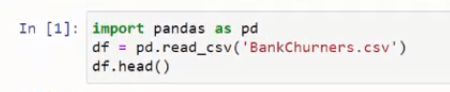To import the dataset, use the pandas read CSV function and save it under the variable df. We can use the head function to access the head (first five rows) of the data just by using the dot head function.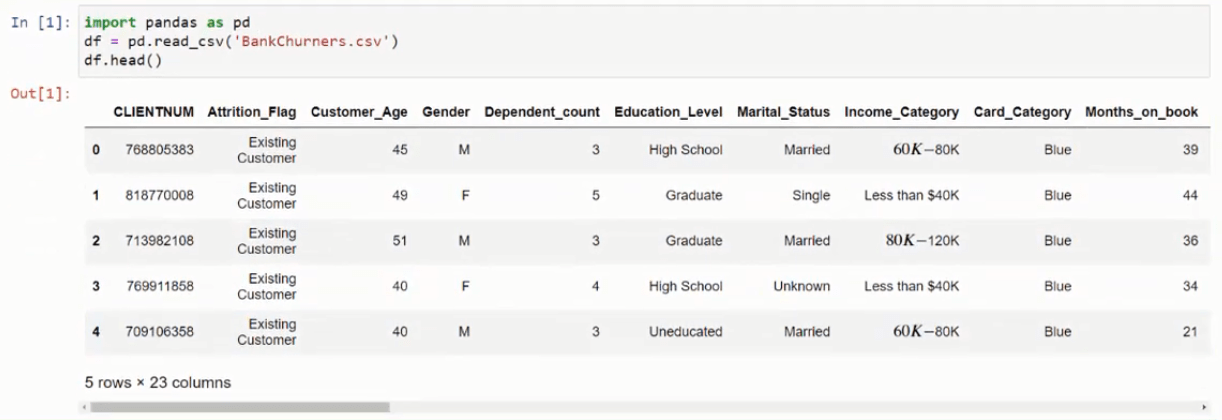So here we have client number, the attribution flag which we’re going to be using for our churn flag, customer age, gender, dependent count, education level, marital status, income level, card category and months on book.

## Syntax

pandas.crosstab(index, columns, values=None, rownames=None, colnames=None, aggfunc=None, margins=False, margins_name=’All’, dropna=True, normalize=False)

To use this function we can specify an index, specify columns, value, row names, aggregation functions, column names and margins and we can also normalize this. We’ll be using the majority of these options in today’s tutorial.

So let’s start answering some of our questions using this pandas function.

## What is the relationship between income and churn?

To answer this question, we’ll use the Income_Category (income level) as the index, and the Attrition_Flag (which tells if someone is an existing customer or they have left the company) as the columns. We’ll also use margins=true to get a total number of customers in each income bracket.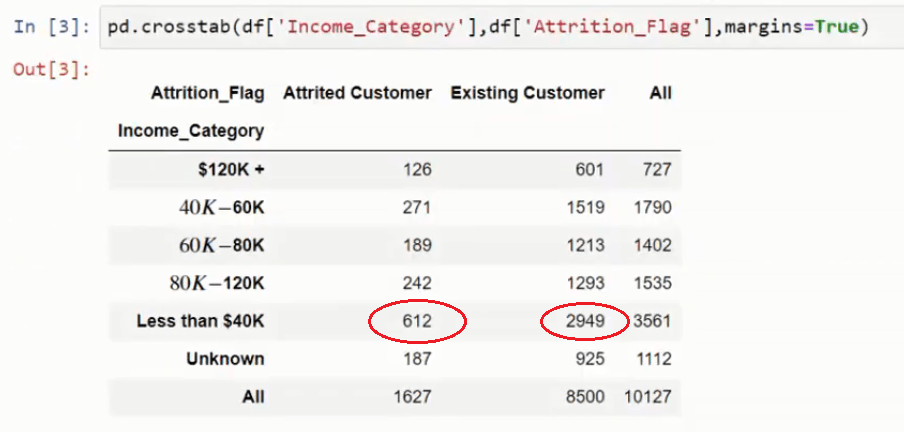The Crosstab function will give us the breakdown of customers at each level of the income along with the total customers.

As you can see, majority of the customers seem to be under the \$40,000 mark.

Let’s add to this a bit more. To see the customer numbers as a percentage, we can use the normalize argument. By adding normalize=true to the Crosstab function, we get all values as a percentage.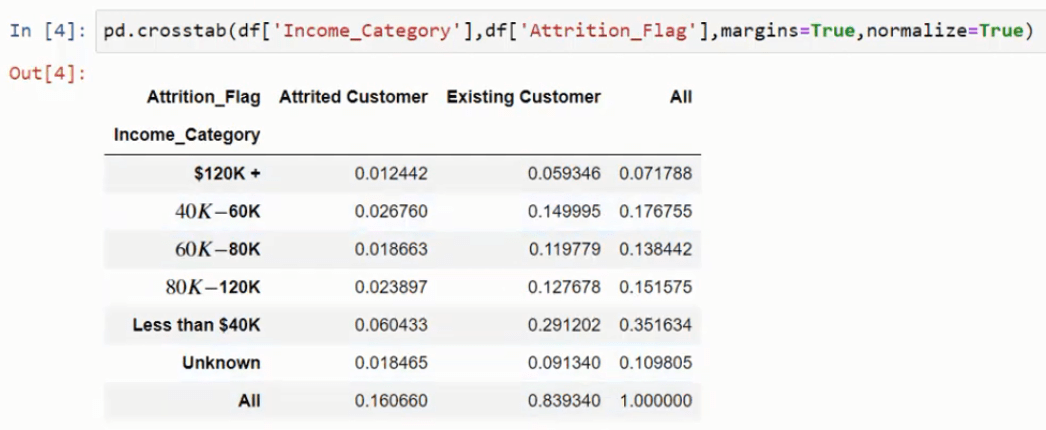We get the number of customers (churned of existing) as a percent of total customers in the system – 10127 in this case. So you can now see that our churn rate is 16% and existing customers are 83% of the total customers in the database.

However, you may want to see the total percentage of customers who have churned and not the total percent of customers who churned in or existing, and the way we can do that is specify where we want our normalization to occur.

We can do this is two ways

• If we normalize by index, the number of customers is shown as a percent of total in each row.
• If we however, normalize by column, the number of customers is shown as a percent of total in each column.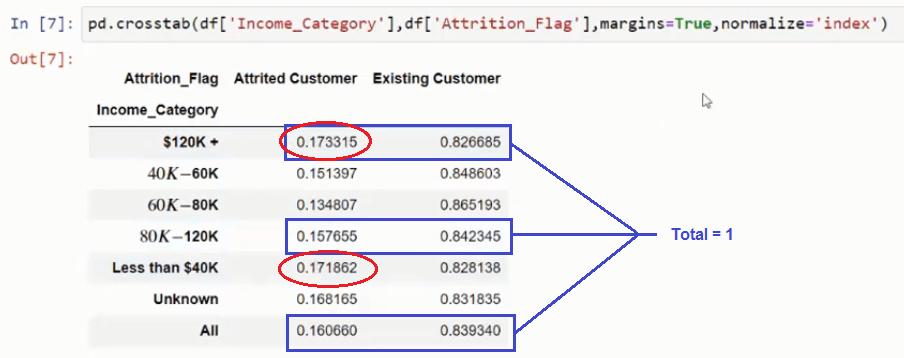Using index we can see that the customers in the income categories above \$120K and less than \$40K have the highest attrition rate.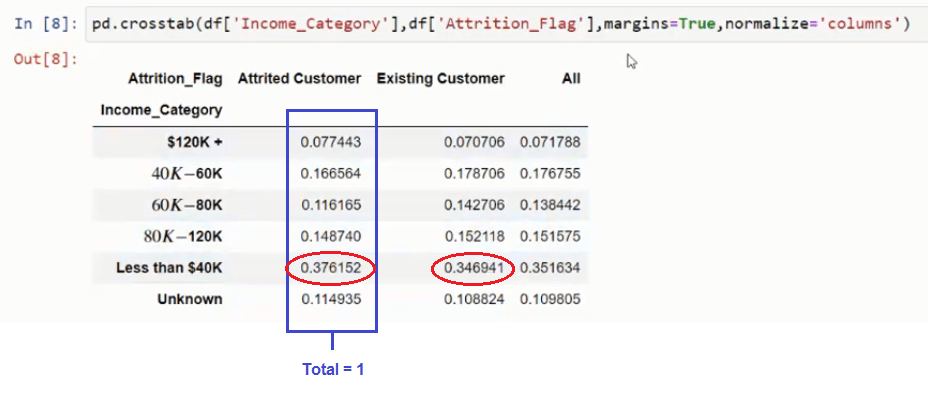Using columns we can see that our biggest customer base in both columns is that of customers with income less than \$40K.

## What is the relationship between education and churn?

For this relationship, continue as in the previous one but use Education_Level as the index and remove the normalize attribute.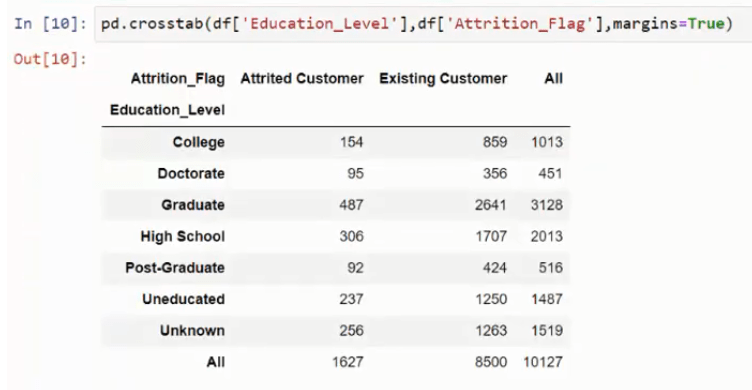## What is the relationship between gender and churn?

By now hopefully you can guess this one. Use Gender as the index to get the customer attribution breakdown for each gender.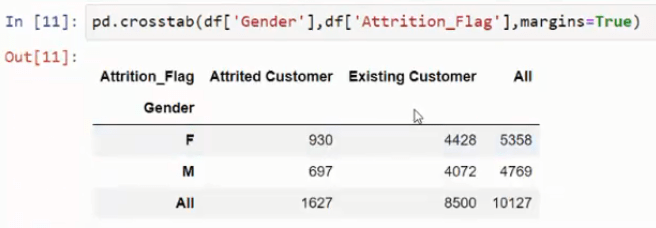You can thus do different analyses by using the margins and the normalizations along the columns or the indexes to get the information you want.

## Of those who churned, what is the average number of months on book by card type?

For this, we start with Card_Category as the index and Attrition_Flag as the column. Then we will also use Months_on_book as the value and mean (average) aggregate-function as our third and fourth attributes to see the average number of months on book instead of the number of customers as in the previous cases.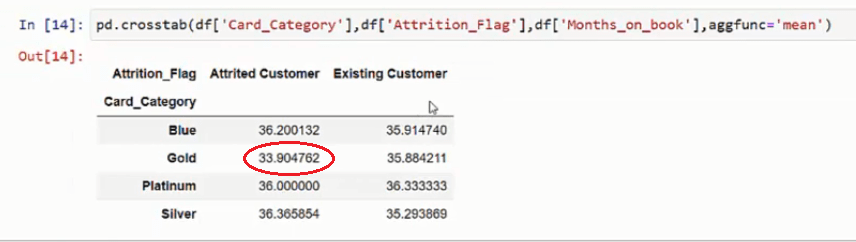This gives us the average number of months that someone is a card customer at the different Card levels. The Gold category seems to lose customers a little bit early since the number of month is the least.

So for the Blue, Platinum and Silver categories, we can think about doing some re-targeting and reaching out to the customers at about 36 months, to see if they’re going to leave the program. Similarly in the Gold category, we may want to reach out to the customers earlier.

Now use the Crosstab function to see what other inferences you can gather from this dataset.

• in
• |
• April 20, 2021#### Author2

Subscribe
Notify of